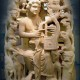Mechanics

# Types of Physical QuantitiesPhysical quantities are those quantities that can be measured. Basically, there are two types of physical quantities (Base quantities or fundamental quantities) and (Derived quantities).
These are quantities that are used to describe the laws of physics. Physical quantities may be divided into six categories.

1. Constant or ratio

Such quantities have the only magnitude, for example, refractive index, dielectric constant, and specific gravity. Such quantities have no units.

1. Scalars

These quantities have magnitude and unit. Some of them may have direction also but vector laws are not applied. Examples are the charge, mass, distance, speed, and current.

1. Vectors

These quantities possess magnitude, unit, and direction. They also follow the triangle law of addition. For example velocity, force, momentum, torque, and displacement.

1. Phasors

These possess magnitude (amplitude) and phase. Try to follows the triangle law. Simple harmonic motion (SHM), waves. AC voltage and AC current are phasors.

1. Tensors

Such quantities do not have any specified direction but have different values in different directions. For example moment of inertia. In anisotropic media even density, refractive index, dielectric constant, electric conductivity, stress, strain, and so on become tensor. A physical quantity that has only one component is called a scalar or a tensor of zero ranks. If it has more than one component but less than or equal to four, it is called a vector or a tensor of rank 1. If the components are greater than four, it is termed as a tensor of a higher rank.

1. Conversion Factors

Some physical quantities convert into another when multiple by a factor. For example, in a wave y = y0 sin (ωt – kx), k is a conversion factor. When k is multiplied by displacement or path difference it generates phases or phase difference. In frequency modulation kEmfc = δ, k converts the voltage into the angle and is termed as a conversion factor. Many other conversion factors can be thought of.
In general, a physical quantity = magnitude x unit. If the unit changes, the magnitude will also change. We apply n1u1 = n2u2.
Physical quantities may be divided into fundamental and derived quantities.
Fundamental Quantities
The quantities that do not depend upon any other quantity are called “fundamental or absolute or basic quantities”.  Initially, only three fundamental quantities –length, mass, and time – were considered. With the development of science, four more physical quantities were added. These are temperature, electric current, luminous intensity, and amount of a substance.

## Physical quantities examples

• Mass
• Length
• Time
• Electric current
• Volume
• Area
• Density
• Force
• Energy
• Power
• Electric flux
• Electric potential
• Speed
• Velocity
• Acceleration
• Momentum
• Torque

Derived Quantities
The physical quantities derived from the fundamental quantities are called derived quantities like velocity, acceleration, force, and momentum.
Related posts:

### Related Articles

1.ricky says:

sup

1.ricky says:

sup
sup
sup
sup
sup
sup
sup
sup
sup
sup
sup
su[p
sup

2.Devils Advocate says:

Mass/Length/Time/Electric current/Volume/Area/Density/Force/Energy/Power/Electric flux/Electric potential/Velocity/Acceleration/Momentum/Torque are NOT Physical quantities – They are Properties & a property is any (macroscopic) observable characteristic of a system (relates to matter ONLY). For example, pressure, volume and density, energy, frequency, entropy, temperature are properties.
The Physical quantities, like power and work, require for their meaningful handling the establishment of systems of units. The quantities and units is relevant to the subject of Mechanics.
The Physical quantities have to have a number accompanied with a unit. Length for example can be in feet, centimeters, or meters… etc, so Length is a observable characteristic of a system (relates to matter ONLY) (property), but 1m Length is Physical quantity